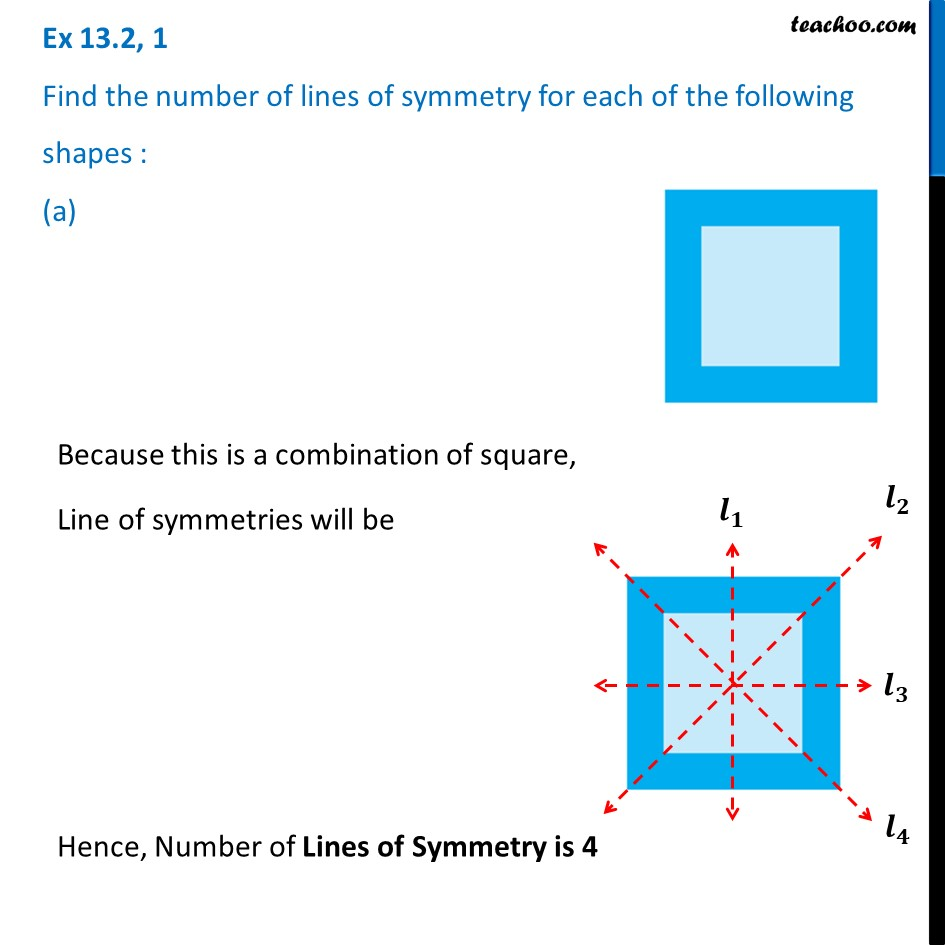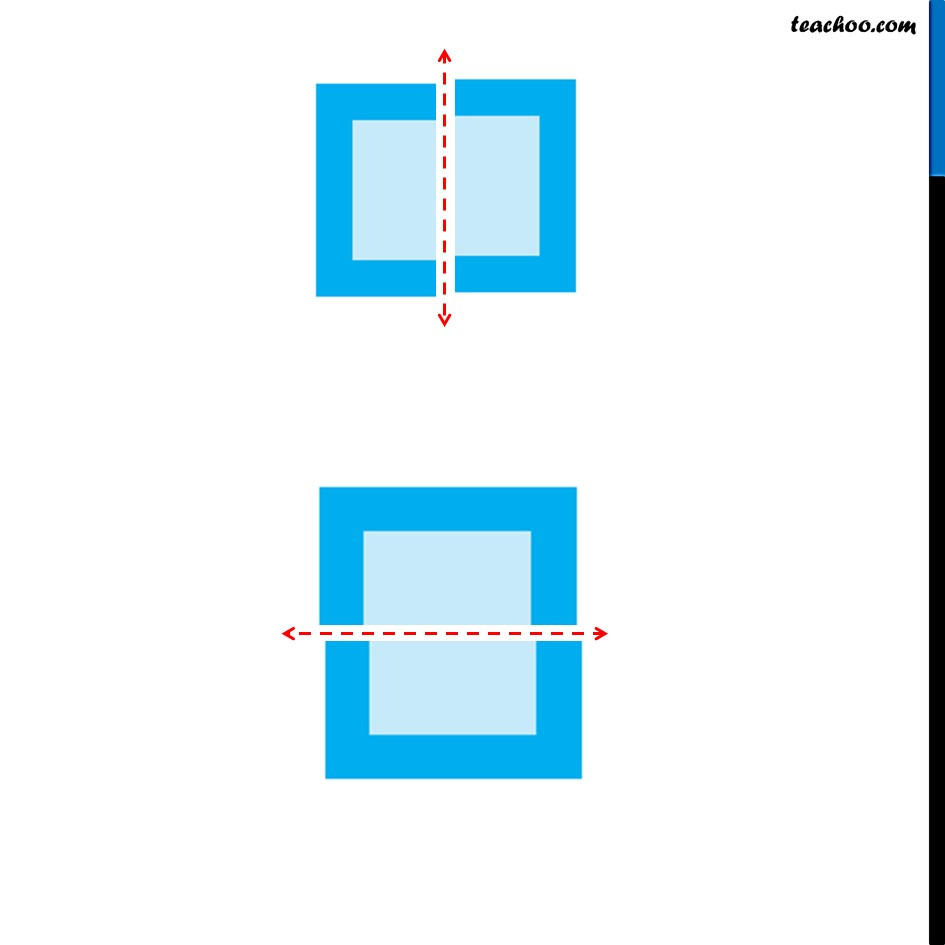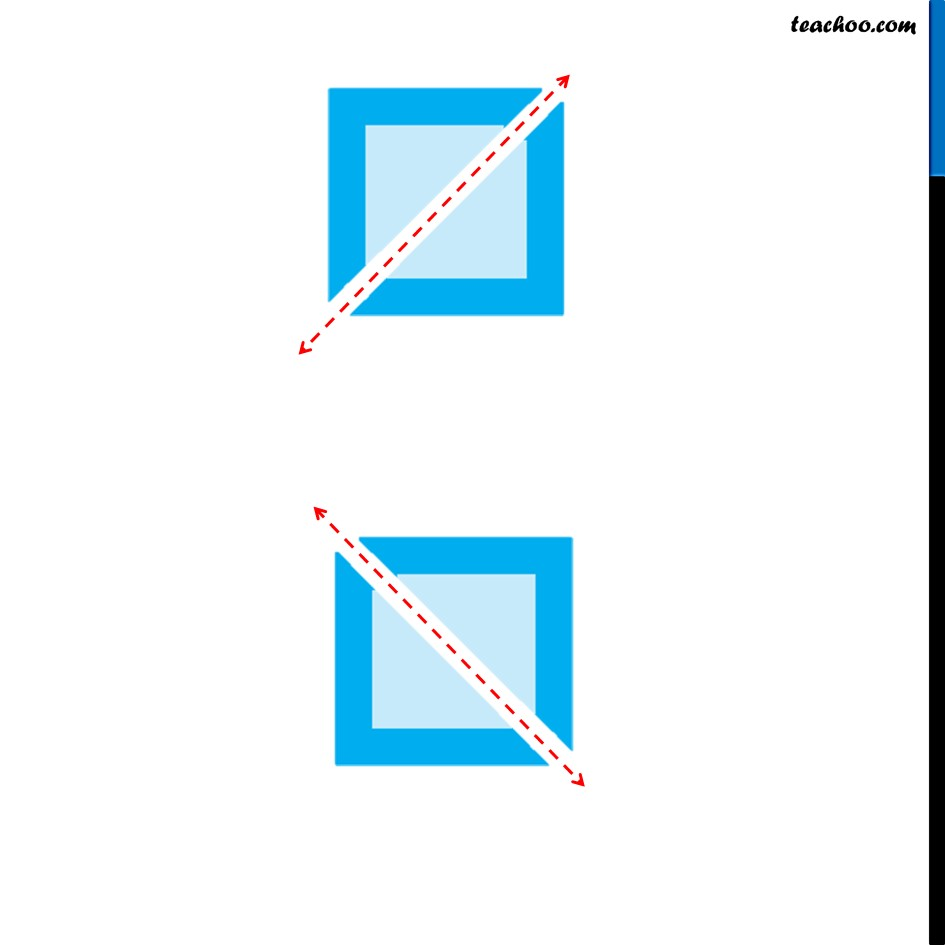1. Chapter 13 Class 6 Symmetry
2. Serial order wise
3. Ex 13.2

Transcript

Ex 13.2, 1 Find the number of lines of symmetry for each of the following shapes : (a) Because this is a combination of square, Line of symmetries will be Hence, Number of Lines of Symmetry is 4

Ex 13.2

Chapter 13 Class 6 Symmetry
Serial order wise